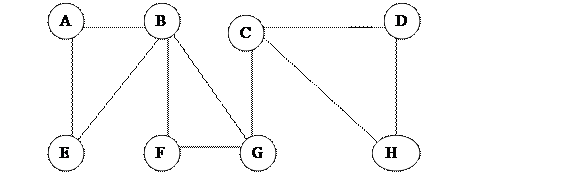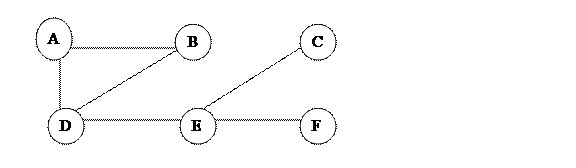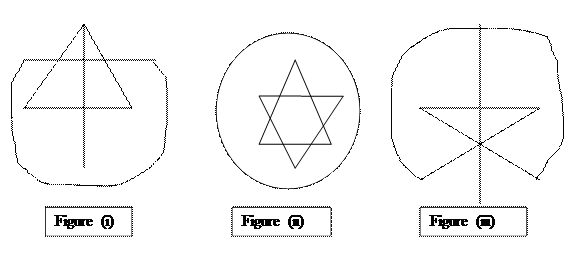# B.Tech Information Technology Assignment 1- Discrete mathematics - Question Paper | lpu

Question 1. Consider the graph in fig one and obtain (a) the degree of every vertex and verify the handshaking lemma
(b) all simple paths from A to G
(c) all trails (Distinct edges) from B to C
(d) d(A,C), The distance from A to C
(e)Diameter (G)Question 2. Consider the graph as shown in the fig one and obtain all the cycles, all cut points and bridges if any.

Question 3. Consider the subsequent graph. obtain the sub graph H(V’,E’) generated by:
(a) V’={B,C,D,E,F};(B) V’={A.C,E,G,H};(C) V’={B,D,E,H};(D) V’={C,F,G,H}
(b) Which of them are isomorphic and which are homomorphic?Question 4. Can a simple graph exists with 15 vertices every of degree five?

Question 5. Consider the multigraph G as shown in the figure (a)Which of them are connected? if they are not obtain the number of connected components .(b)which are cycle free .if they are not obtain the number of cycles (c)Which are loop free and Which are simple graphs?Question 6. Show that graph g with n vertices must have n-1 edges ?

Question 7. Draw 2 3-regular graphs with eight vertices ?

Question 8. consider every graph in G .Find the euler path or euler circuit if exists?and if does not why?
(see image in attachment )

Question 9. Consider every graph in G. obtain the Hamiltonian Path or circuit if exists and if does not why???

Question 10. Consider the complete graph Kn
(a) Find the no of m edges in Kn
(b) Find the degree of every vertex in Kn
(c) Find those values of n for which Kn is regular

Question 11. Suppose that G and G* ARE HOMOMORPHIC. Show that G is Eulerian if and only if G* is Eulerian.